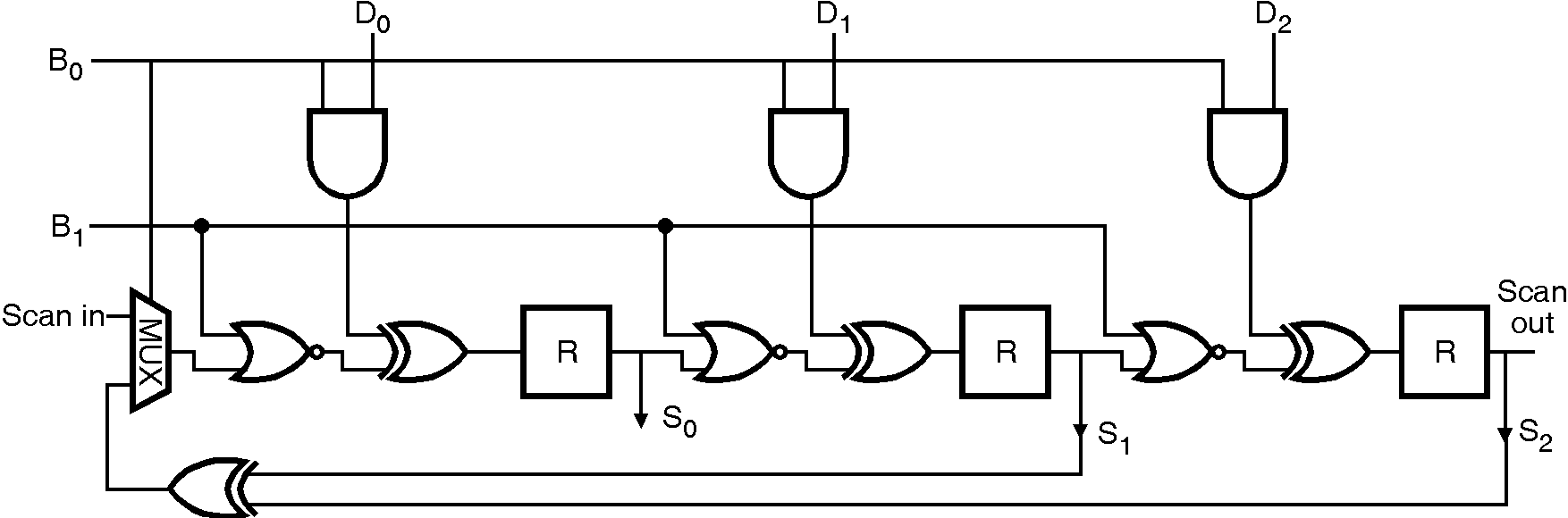﻿ VLSI Design for Testability

Built-in Logic Block Observer (BILBO) :

In this method, signature analysis along with scan path is used. It is aimed at integrated modular and bus-oriented systems, such as microprocessor and similar circuits. Below figure the BILBO is controlled by two signals, B1 and B2, which define the modes. In the normal mode, B1 = B2 = 1 and the storage elements are used independently by the circuit. In the Test1 mode B1 = B2 = 0 and the storage elements are configured as a scan path, all storage elements being connected as a serial shift register. Test vectors are then applied to the scan-in input and responses shifted out at the scan path output. The analysis of data is then similar to that for a simple scan path test. In the Test2 mode B1 = 1, B2 = 0 and the circuit is then configured in a LFSR mode and can be used either as a polynomial divider to compact data or as a random test pattern generator. In the final mode, B1 = 0, B2 = 1 which resets the BILBO.